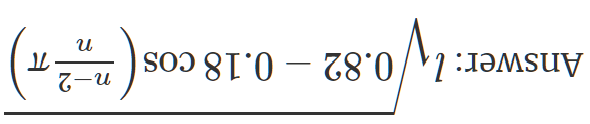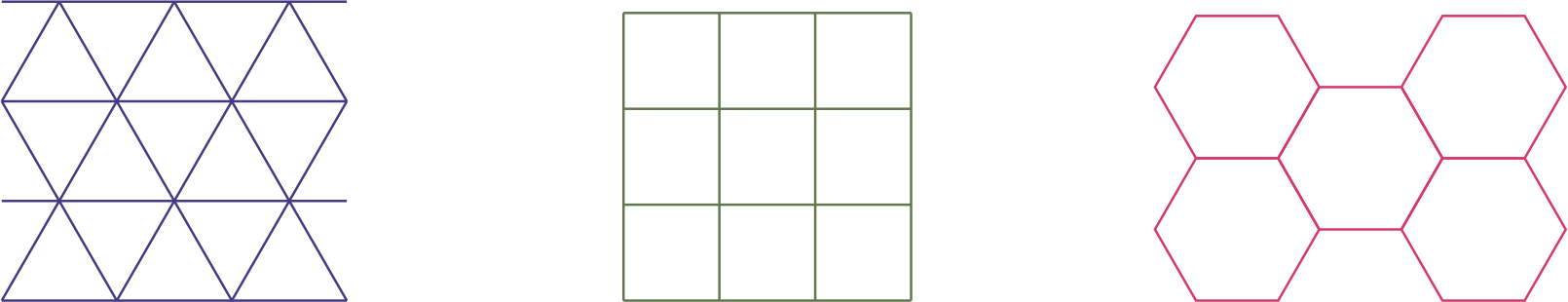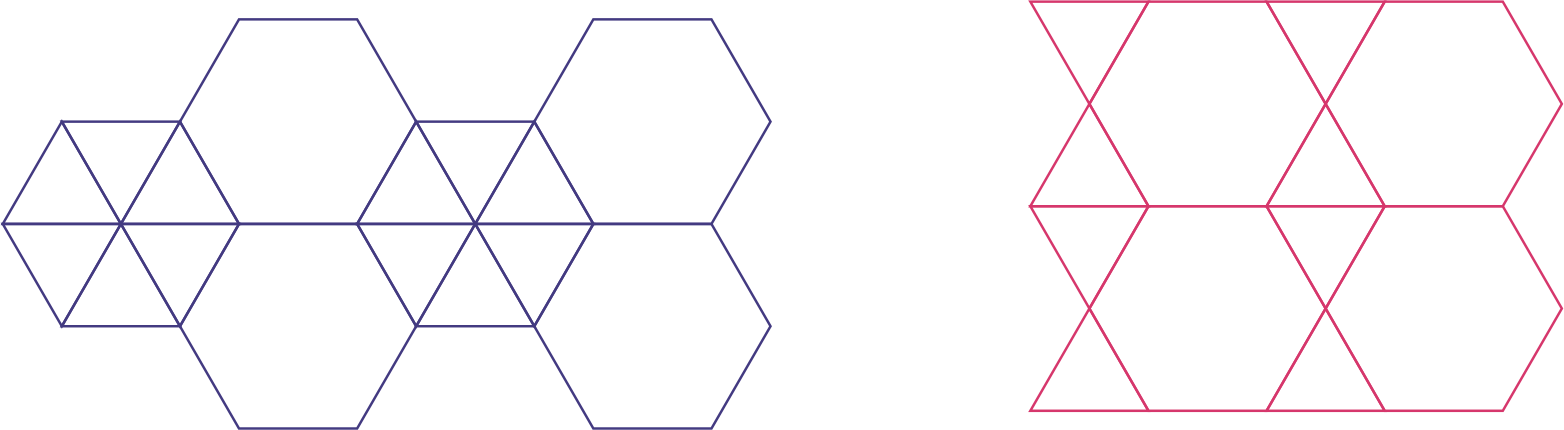# Colouring for mindfulness

Florian Bouyer explains the beautiful geometry behind his mathematical colouring-in designs.Imagine three mice equally distanced from each other, ie at the vertices of an equilateral triangle. If at the same time, all three mice start chasing their neighbour clockwise, then each of their paths would be a logarithmic curve. But this is rather hard to draw, especially if we want to restrict ourselves to only using a ruler.

Instead, let us imagine that the mice can only run in a straight line and need to stop to reassess their direction. If at a given stage we draw their intended path, and assume that the mice cover a tenth of the distance to the next mouse before stopping and reassessing their direction, we get the picture below. While these pictures have been drawn using straight lines only, we see three logarithmic spirals emerging:

But why stop there? Why not start with $4$, $5$ or $n$ mice on the vertices of a regular square, pentagon or $n$-gon? The following instructions show a very algorithmic approach to drawing these patterns:

• Draw a regular $n$-gon, each side having length $l$.
• Going clockwise (or anticlockwise) along the vertices, mark the point that is at distance $0.1l$ on the side next to the vertex. (Feel free to experiment with different values such as $0.2l$, $0.13l$ etc.)
• Join all the points together to make a regular $n$-gon with smaller side length.
• Repeat the process with the new $n$-gon.
Question: What is the side length of the new $n$-gon you drew in terms of $n$ and $l$?Here are some of these basic shapes:

## Tiling

While in themselves, the basic shapes can be fun to colour in, more amazing pictures emerge when these basic shapes are put together through tiling. Tiling simply means putting geometric shapes—these are our tiles—down on a flat surface such that they do not overlap or leave any gaps. To keep thing simple, we will only explore edge-to-edge tilings: tilings where each edge of each tile fully touches the edge of another tile. Therefore any two tiles can either:

• share an edge (which we now refer to as an edge of the tiling),
• share a vertex (which we now refer to as a vertex of the tiling), or
• be completely disjoint.

We first explore regular tilings: tilings where all the tiles are the same regular $n$-gon.

## How many regular tilings are there?

A theorem which has been known since antiquity tell us that:

In ye Euclidean plane there are only three regular tilings.

These are:From left to right: equilateral triangles 6 at each vertex; squares 4 at each vertex; and regular hexagons 3 at each vertex.

A quick sketch of the proof is:

• As we are dealing with only one regular polygon, all the vertices of the tiling have the same configuration, so let us pick an arbitrary vertex.
• We must have at least three tiles at that vertex, all made from the same regular $n$-gon.
• Given that the interior angle of a regular $n$-gon is $(n-2)\pi/n$, if $n\geq 7$ we have that the interior angle of $3$ $n$-gons is $\geq 3\times 5\pi/7 > 2\pi$, ie the tiles overlap.
• Therefore, we just need to check for which $n \in \{3, 4, 5, 6\}$ we have an integer $k$ such that $k (n-2)\pi/n = 2\pi$, ie $(n-2)k = 2n$. This leads us to the pairs $(n,k) \in \{(3,6), (4,4), (6,3)\}$.

Combining a regular tiling with the appropriate $n$-mice problem produces more interesting drawings to colour in, such as these triangular and hexagonal tilings:## Tilings of different regular $n$-gons

Of course, we can drop the restrictions that all the tiles are the same and look at tilings where the tiles are regular, but different, $n$-gons. To study these, we look at what could be happening at a given vertex of the tiling. As we know that the interior angle of a regular $n$-gon is $\frac{n-2}{n}\pi = \left(1-\frac{2}{n}\right)\pi,$ if we have $q$ regular polygons with $p_1, p_2, \dots, p_q$ sides then we need that their total interior angle adds up to $2\pi$, ie $\left[q-2\left(\frac{1}{p_1}+\dots+\frac{1}{p_q}\right)\right]\pi = 2\pi.$ So a vertex of a tiling is only possible if there exists $q$ numbers $p_1, \dots, p_q$ such that $\frac{1}{p_1}+\dots+\frac{1}{p_q} = \frac{q-2}{2}.$ It turns out that this condition is restrictive enough to give only 17 different possibilities, of which $4$ give two different configurations. For example, $(3,3,3,4,4)$ and $(3,3,4,3,4)$ both work (you can check!) but the former represents (going round the vertex) 3 triangles followed by 2 squares, while the latter represents 2 triangles followed by a square, a third triangle and a second square.

Once we know the 21 different vertex configurations, we can go through and combine them to see if they can be extended to a full plane tiling! For example, taking the vertex configuration $(3,3,3,3,3,3)$ (six triangles around a vertex, which we already have seen) and $(3,3,6,6)$ (two triangles followed by two hexagons) we can create the tiling here on the left:On the left: $(3,3,3,3,3,3)$ and $(3,3,6,6)$; on the right: $(3,3,6,6)$ and $(3,6,3,6)$.

We could also take the vertex configuration $(3,3,6,6)$ and $(3,6,3,6)$ (triangle, hexagon, triangle, hexagon) to get the tiling on the right. Both of these tilings have two different configurations for their vertices and so are examples of a 2-uniform tiling. In general, a $k$-uniform tiling is a tiling composed of regular $n$-gons with exactly $k$ different vertex configurations. Filling in the tiling on the left with the $3$-mice and $6$-mice problem, we can get this:Note, however, that not all vertex configurations can appear in a tiling. For example, $(5,5,10)$ is a possible configuration, but it is the only configuration that includes both a pentagon and decagon, and also the only configuration that includes at least two pentagons. So if we wanted to extend this to a full tiling, we can only use vertex configuration $(5,5,10)$, but then we can see (with some thought) that at one point we need a tile to be both a pentagon and a decagon at the same time.

## The hyperbolic plane

While we could go on exploring tilings in the Euclidean plane (and you are very much encouraged to do so), it is not the only world we can explore. Let’s look at the hyperbolic plane; in particular, the Poincaré disk model.

The hyperbolic plane was discovered when mathematicians were trying to show that Euclid’s fifth axiom,

Given a line $l$ and a point $P$, there exists a unique line parallel to $l$ going through $P$.

depended on the previous four axioms. This turns out not to be possible, because we can construct a geometric world where the first four axioms of Euclid are true, but the fifth one is replaced by

Given a line $l$ and a point $P$, there exists at least two lines parallel to $l$ going through $P$.

Concretely, we define the Poincaré disk as follows:

• The whole model takes place inside a unit circle $C$ drawn on the Euclidean plane
• Points in the Poincaré disk are Euclidean points inside $C$
• Straight lines in the Poincaré disk are (Euclidean) arcs of circles perpendicular to $C$ (ie circles intersecting $C$ at angles of $\pi/2$), plus any (Euclidean) lines going through the centre of $C$ (which can be taken as the arc of a circle with infinite radius)

The advantage of the Poincaré disk model is that the whole space is contained in a finite Euclidean space, so we can visualise all of it. This said, as the boundary of $C$ represents distance at infinity in the Poincaré disk model, the same shape (ie with fixed hyperbolic side length) will appear smaller (in the Euclidean sense) the closer to the boundary of $C$ it is.

Since we have defined the notion of points and lines in hyperbolic geometry, we can draw $n$-gons and define the notion of tilings in the same way we do in Euclidean geometry. If we look at tiling in the hyperbolic plane, then we get a different picture than in the Euclidean setting.

## How many regular tilings are there in the hyperbolic plane?

In the hyperbolic plane, the angles of a triangle always add up to strictly less than $\pi$ (this is a consequence of the different fifth axiom). Once we know this, we see that the interior angle of a regular $n$-gon can be any value strictly less than $(n-2)\pi/n$. It takes a fair bit of work to show that given an angle $0<\alpha <(n-2)\pi/n$, we can draw a hyperbolic $n$-gon with interior angles $\alpha$. But given this fact, if we fix $n$ then we can fit $k$ $n$-gons round a vertex as long as $\frac{2}{k} < \frac{n-2}{n} \implies k > \frac{2n}{n-2}.$ Hence for any $n$-gon,

There are infinitely many regular tilings in the hyperbolic plane.

As well as tilings, we can see that the $n$-mice problem also translates into the hyperbolic plane, therefore by once again combining the two we can create some nice drawings to be coloured in.

On the front cover of the magazine, we have a tiling of 8 triangles meeting at a vertex, the view being centred on a tile:and on the rear cover, we have a tiling of 8 triangles meeting at a vertex, the view being centred on a vertex of the tiling:and a tiling of 6 squares meeting at a vertex, the view being centred on a vertex of the tiling:You can find high-resolution versions, and more tilings, on my website.

## Draw these pictures yourself

Hopefully, the above has intrigued you into exploring and making your own kind of drawings. If you want, they can all be done with a pencil, ruler (to draw and measure distances) and compass—even the lines in the Poincaré disk model (Compass and Straightedge in the Poincaré Disk by Chaim Goodman-Strauss tells you how). But this is a very slow process so the drawings included here were done by computer.

Originally, I produced the drawings using the free program Cinderella.2. This is a geometry program and can draw regular $n$-gons, both on the Euclidean plane and the hyperbolic plane. It also has the ability to understand and apply various transformations to shapes drawn.

In practice, this can be a bit fiddly, and so these drawings can (and have) been produced by using the TikZ package in LaTeX. This has more of an algorithmic flavour and you cannot see what you are producing until you compile your code, but can be less computer intensive and the code can easily be copied. The procedure is slightly different and for the moment only works in Euclidean geometry.

Check back in a few weeks to find a guide to drawing tilings with the $n$-mice problem using both Cinderella.2 and TikZ.